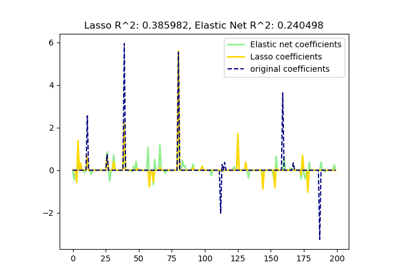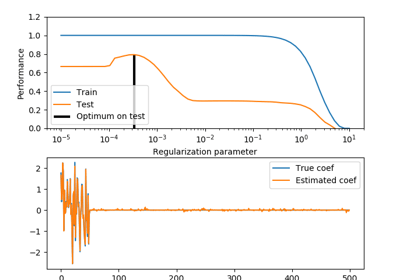/scikit-learn

# sklearn.linear_model.ElasticNet

class sklearn.linear_model.ElasticNet(alpha=1.0, l1_ratio=0.5, fit_intercept=True, normalize=False, precompute=False, max_iter=1000, copy_X=True, tol=0.0001, warm_start=False, positive=False, random_state=None, selection=’cyclic’) [source]

Linear regression with combined L1 and L2 priors as regularizer.

Minimizes the objective function:

1 / (2 * n_samples) * ||y - Xw||^2_2
+ alpha * l1_ratio * ||w||_1
+ 0.5 * alpha * (1 - l1_ratio) * ||w||^2_2


If you are interested in controlling the L1 and L2 penalty separately, keep in mind that this is equivalent to:

a * L1 + b * L2


where:

alpha = a + b and l1_ratio = a / (a + b)


The parameter l1_ratio corresponds to alpha in the glmnet R package while alpha corresponds to the lambda parameter in glmnet. Specifically, l1_ratio = 1 is the lasso penalty. Currently, l1_ratio <= 0.01 is not reliable, unless you supply your own sequence of alpha.

Read more in the User Guide.

Parameters: alpha : float, optional Constant that multiplies the penalty terms. Defaults to 1.0. See the notes for the exact mathematical meaning of this parameter.alpha = 0 is equivalent to an ordinary least square, solved by the LinearRegression object. For numerical reasons, using alpha = 0 with the Lasso object is not advised. Given this, you should use the LinearRegression object. l1_ratio : float The ElasticNet mixing parameter, with 0 <= l1_ratio <= 1. For l1_ratio = 0 the penalty is an L2 penalty. For l1_ratio = 1 it is an L1 penalty. For 0 < l1_ratio < 1, the penalty is a combination of L1 and L2. fit_intercept : bool Whether the intercept should be estimated or not. If False, the data is assumed to be already centered. normalize : boolean, optional, default False This parameter is ignored when fit_intercept is set to False. If True, the regressors X will be normalized before regression by subtracting the mean and dividing by the l2-norm. If you wish to standardize, please use sklearn.preprocessing.StandardScaler before calling fit on an estimator with normalize=False. precompute : True | False | array-like Whether to use a precomputed Gram matrix to speed up calculations. The Gram matrix can also be passed as argument. For sparse input this option is always True to preserve sparsity. max_iter : int, optional The maximum number of iterations copy_X : boolean, optional, default True If True, X will be copied; else, it may be overwritten. tol : float, optional The tolerance for the optimization: if the updates are smaller than tol, the optimization code checks the dual gap for optimality and continues until it is smaller than tol. warm_start : bool, optional When set to True, reuse the solution of the previous call to fit as initialization, otherwise, just erase the previous solution. See the Glossary. positive : bool, optional When set to True, forces the coefficients to be positive. random_state : int, RandomState instance or None, optional, default None The seed of the pseudo random number generator that selects a random feature to update. If int, random_state is the seed used by the random number generator; If RandomState instance, random_state is the random number generator; If None, the random number generator is the RandomState instance used by np.random. Used when selection == ‘random’. selection : str, default ‘cyclic’ If set to ‘random’, a random coefficient is updated every iteration rather than looping over features sequentially by default. This (setting to ‘random’) often leads to significantly faster convergence especially when tol is higher than 1e-4. coef_ : array, shape (n_features,) | (n_targets, n_features) parameter vector (w in the cost function formula) sparse_coef_ : scipy.sparse matrix, shape (n_features, 1) | (n_targets, n_features) sparse representation of the fitted coef_ intercept_ : float | array, shape (n_targets,) independent term in decision function. n_iter_ : array-like, shape (n_targets,) number of iterations run by the coordinate descent solver to reach the specified tolerance.

ElasticNetCV
Elastic net model with best model selection by cross-validation.
SGDRegressor
implements elastic net regression with incremental training.
SGDClassifier
implements logistic regression with elastic net penalty (SGDClassifier(loss="log", penalty="elasticnet")).

#### Notes

To avoid unnecessary memory duplication the X argument of the fit method should be directly passed as a Fortran-contiguous numpy array.

#### Examples

>>> from sklearn.linear_model import ElasticNet
>>> from sklearn.datasets import make_regression
>>>
>>> X, y = make_regression(n_features=2, random_state=0)
>>> regr = ElasticNet(random_state=0)
>>> regr.fit(X, y)
ElasticNet(alpha=1.0, copy_X=True, fit_intercept=True, l1_ratio=0.5,
max_iter=1000, normalize=False, positive=False, precompute=False,
random_state=0, selection='cyclic', tol=0.0001, warm_start=False)
>>> print(regr.coef_)
[18.83816048 64.55968825]
>>> print(regr.intercept_)
1.451...
>>> print(regr.predict([[0, 0]]))
[1.451...]


#### Methods

 fit(X, y[, check_input]) Fit model with coordinate descent. get_params([deep]) Get parameters for this estimator. path(X, y[, l1_ratio, eps, n_alphas, …]) Compute elastic net path with coordinate descent predict(X) Predict using the linear model score(X, y[, sample_weight]) Returns the coefficient of determination R^2 of the prediction. set_params(**params) Set the parameters of this estimator.
__init__(alpha=1.0, l1_ratio=0.5, fit_intercept=True, normalize=False, precompute=False, max_iter=1000, copy_X=True, tol=0.0001, warm_start=False, positive=False, random_state=None, selection=’cyclic’) [source]
fit(X, y, check_input=True) [source]

Fit model with coordinate descent.

Parameters: X : ndarray or scipy.sparse matrix, (n_samples, n_features) Data y : ndarray, shape (n_samples,) or (n_samples, n_targets) Target. Will be cast to X’s dtype if necessary check_input : boolean, (default=True) Allow to bypass several input checking. Don’t use this parameter unless you know what you do.

#### Notes

Coordinate descent is an algorithm that considers each column of data at a time hence it will automatically convert the X input as a Fortran-contiguous numpy array if necessary.

To avoid memory re-allocation it is advised to allocate the initial data in memory directly using that format.

get_params(deep=True) [source]

Get parameters for this estimator.

Parameters: deep : boolean, optional If True, will return the parameters for this estimator and contained subobjects that are estimators. params : mapping of string to any Parameter names mapped to their values.
static path(X, y, l1_ratio=0.5, eps=0.001, n_alphas=100, alphas=None, precompute=’auto’, Xy=None, copy_X=True, coef_init=None, verbose=False, return_n_iter=False, positive=False, check_input=True, **params) [source]

Compute elastic net path with coordinate descent

The elastic net optimization function varies for mono and multi-outputs.

1 / (2 * n_samples) * ||y - Xw||^2_2
+ alpha * l1_ratio * ||w||_1
+ 0.5 * alpha * (1 - l1_ratio) * ||w||^2_2


(1 / (2 * n_samples)) * ||Y - XW||^Fro_2
+ alpha * l1_ratio * ||W||_21
+ 0.5 * alpha * (1 - l1_ratio) * ||W||_Fro^2


Where:

||W||_21 = \sum_i \sqrt{\sum_j w_{ij}^2}


i.e. the sum of norm of each row.

Read more in the User Guide.

Parameters: X : {array-like}, shape (n_samples, n_features) Training data. Pass directly as Fortran-contiguous data to avoid unnecessary memory duplication. If y is mono-output then X can be sparse. y : ndarray, shape (n_samples,) or (n_samples, n_outputs) Target values l1_ratio : float, optional float between 0 and 1 passed to elastic net (scaling between l1 and l2 penalties). l1_ratio=1 corresponds to the Lasso eps : float Length of the path. eps=1e-3 means that alpha_min / alpha_max = 1e-3 n_alphas : int, optional Number of alphas along the regularization path alphas : ndarray, optional List of alphas where to compute the models. If None alphas are set automatically precompute : True | False | ‘auto’ | array-like Whether to use a precomputed Gram matrix to speed up calculations. If set to 'auto' let us decide. The Gram matrix can also be passed as argument. Xy : array-like, optional Xy = np.dot(X.T, y) that can be precomputed. It is useful only when the Gram matrix is precomputed. copy_X : boolean, optional, default True If True, X will be copied; else, it may be overwritten. coef_init : array, shape (n_features, ) | None The initial values of the coefficients. verbose : bool or integer Amount of verbosity. return_n_iter : bool whether to return the number of iterations or not. positive : bool, default False If set to True, forces coefficients to be positive. (Only allowed when y.ndim == 1). check_input : bool, default True Skip input validation checks, including the Gram matrix when provided assuming there are handled by the caller when check_input=False. **params : kwargs keyword arguments passed to the coordinate descent solver. alphas : array, shape (n_alphas,) The alphas along the path where models are computed. coefs : array, shape (n_features, n_alphas) or (n_outputs, n_features, n_alphas) Coefficients along the path. dual_gaps : array, shape (n_alphas,) The dual gaps at the end of the optimization for each alpha. n_iters : array-like, shape (n_alphas,) The number of iterations taken by the coordinate descent optimizer to reach the specified tolerance for each alpha. (Is returned when return_n_iter is set to True).

#### Notes

For an example, see examples/linear_model/plot_lasso_coordinate_descent_path.py.

predict(X) [source]

Predict using the linear model

Parameters: X : array_like or sparse matrix, shape (n_samples, n_features) Samples. C : array, shape (n_samples,) Returns predicted values.
score(X, y, sample_weight=None) [source]

Returns the coefficient of determination R^2 of the prediction.

The coefficient R^2 is defined as (1 - u/v), where u is the residual sum of squares ((y_true - y_pred) ** 2).sum() and v is the total sum of squares ((y_true - y_true.mean()) ** 2).sum(). The best possible score is 1.0 and it can be negative (because the model can be arbitrarily worse). A constant model that always predicts the expected value of y, disregarding the input features, would get a R^2 score of 0.0.

Parameters: X : array-like, shape = (n_samples, n_features) Test samples. For some estimators this may be a precomputed kernel matrix instead, shape = (n_samples, n_samples_fitted], where n_samples_fitted is the number of samples used in the fitting for the estimator. y : array-like, shape = (n_samples) or (n_samples, n_outputs) True values for X. sample_weight : array-like, shape = [n_samples], optional Sample weights. score : float R^2 of self.predict(X) wrt. y.
set_params(**params) [source]

Set the parameters of this estimator.

The method works on simple estimators as well as on nested objects (such as pipelines). The latter have parameters of the form <component>__<parameter> so that it’s possible to update each component of a nested object.

Returns: self
sparse_coef_

sparse representation of the fitted coef_

## Examples using sklearn.linear_model.ElasticNetLasso and Elastic Net for Sparse SignalsTrain error vs Test error